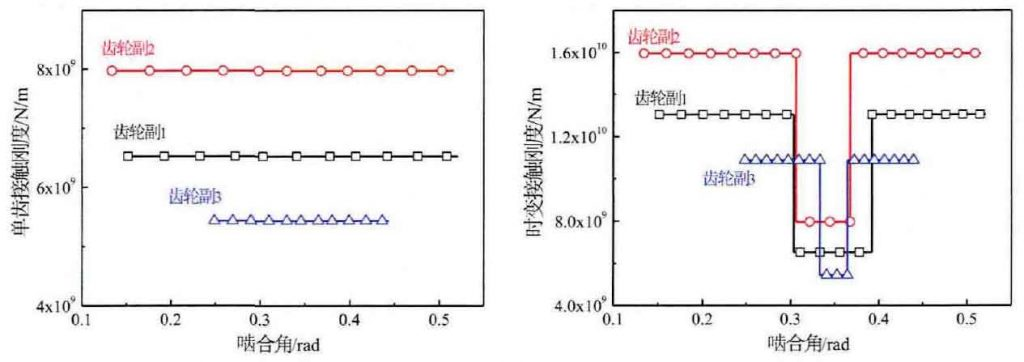# Example simulation and parameter influence analysis of meshing contact stiffness of gear tooth surface

The parameters of gear pair are selected as the calculation object, and the contact stiffness of gear pair under Yang sun model and semi empirical formula is calculated. In Fig. 1 (a), the contact stiffness curves of three pairs of single teeth with different tooth width effects (independent and load) are shown. The numerical superposition effect of contact stiffness is carried out according to the series parallel stiffness relationship of single double teeth meshing area, and the corresponding time-varying contact stiffness curves are drawn (Fig. 1 (b)). Combined with the constant mode of the contact stiffness of the formula, it is consistent with the continuous horizontal line of the contact stiffness of each stage shown in the figure.(a) Single tooth contact stiffness (b) Time varying contact stiffness

Taking the gear pair 1 as an example, the load ratio and load distribution curve calculated by the formula are shown in Fig. 2. Fig. 2 (a) shows that the load ratio curve in the double meshing area presents a constant slope straight-line rising and falling mode of y = B ± KX, and in the single meshing area it is expressed as a constant value curve mode of load ratio equal to 1. The formula shows that the load distribution is proportional to the load ratio and normal load form. Fig. 2 (b) shows that the load distribution increases with the increase of torque, and the load distribution and load ratio distribution form are consistent. However, the load increment is not equal at the same meshing point with the same torque increase.